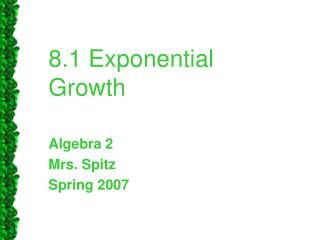DownloadDownload Presentation8.1 Exponential Growth

# 8.1 Exponential Growth

Download Presentation## 8.1 Exponential Growth

- - - - - - - - - - - - - - - - - - - - - - - - - - - E N D - - - - - - - - - - - - - - - - - - - - - - - - - - -
##### Presentation Transcript

1. 8.1 Exponential Growth Algebra 2 Mrs. Spitz Spring 2007

2. Objective • Graph exponential growth functions • Use exponential growth functions to model real-life situations, such as Internet growth. Assignment • Class Activity – Graphing Exponential Functions

3. Graphing Exponential Growth Functions • An exponential function involves the expression bx where base b is a positive number other than 1. In this lesson, you will study exponential functions for which b > 1. To see the basic shape of the graph of an exponential function such as f(x) = 2x, you can make a table of values and plot points as shown on the next slide.

4. Table of values/graph

5. Notice the end behavior of the graph. As which means that the graph moves up to the right. As which means that the graph has the line y = 0 as an asymptote. An asymptote is a line that a graph approaches as you move away from the origin.

6. YOU NEED THIS TO DO THE WORK! • The graph passes through the point (0, a). That is, the y-intercept is a. • The x-axis is an asymptote of the graph. • The domain is all real numbers. • The range is y > 0 if a > 0 and y< 0 if a < 0

7. There is no a ● 4x, so the y intercept is (0, 1) The x-axis is an asymptote of the graph. The domain is all real numbers. Range is y > 0 because a > 0. When x = 1, y = 4 Ex. 1: Graph:

8. There is no a ● 2x-3 +1, so the y intercept is (0, 1) The x-axis is an asymptote of the graph. The domain is all real numbers. Range is y > 0 because a > 0. When x = 1, y = 4 Ex. 2: Graph:

9. To graph a general exponential function: Begin by sketching the graph of y = abx . Then translate the graph horizontally by h units and vertically by k units.

10. Ex. 2: Graphing a general exponential function First graph the first function then move it over 1 place to the right and then four down.

11. On a TI-83 or 84 press y= insert 1 divided by 2 times 3 power x press graph press 2nd graph to get the table of values.

12. Ex. 3: All of the properties of rational exponents apply to real exponents as well. Lucky you! Simplify: Recall the product of powers property, am an = am+n

13. Ex. 4: All of the properties of rational exponents apply to real exponents as well. Lucky you! Simplify: Recall the power of a power property, (am)n= amn

14. Definition of Exponential Function • An equation of the form y = ax, where a > 0 and a ≠ 1, is called an exponential function.

15. Several exponential functions have been graphed at the right. Compare the graphs of functions where a > 1 to those where a < 1. Notice that when a > 1, the value of y increases as the value of x increases. When a < 1, the value of y decreases as the value of x increases. What do they look like graphed?

16. Property of Equality for Exponential Functions • The following property is very useful when solving equations involving exponential functions. • Suppose a is a positive number other than 1. Then: If and only if x1 = x2 Notice that a cannot equal 1. Since 1x = 1 for any real number, for any choice of x1 and x2

17. Ex. 5: Solve